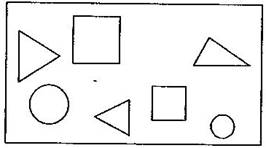# See the figure and find the ratio of:(a)Number of triangles to the number of circles inside the rectangle.(b)Number of squares to all the figures inside the rectangle.(c)Number of circles to all the figures inside the rectangle.

Dear student

Regards

• 0
What are you looking for?